# Reading analog scales

## Instrumentation and Process Control

• #### Question 1

Interpret the pressure measurement displayed by this gauge mechanism, assuming a gauge accuracy of $\pm$ 1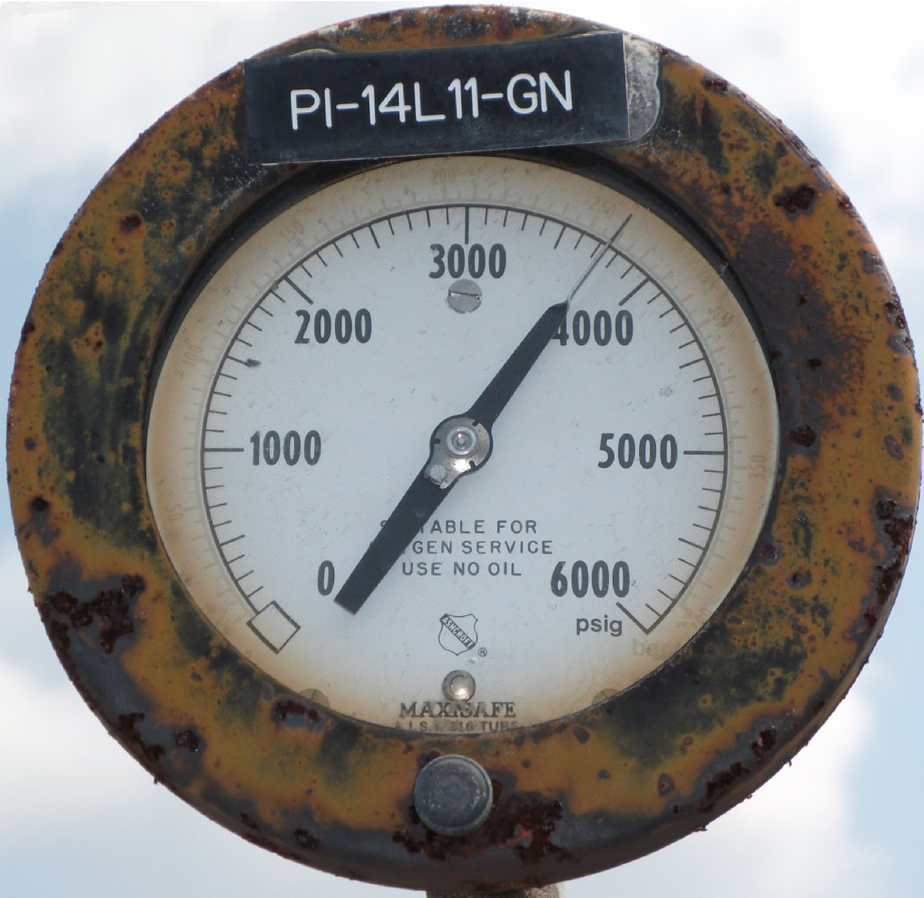How low and how high could this pressure actually be, given the stated accuracy of this gauge?

Reveal answer
• #### Question 2

Interpret the pressure measurement displayed by this gauge mechanism, assuming a gauge accuracy of $\pm$ 2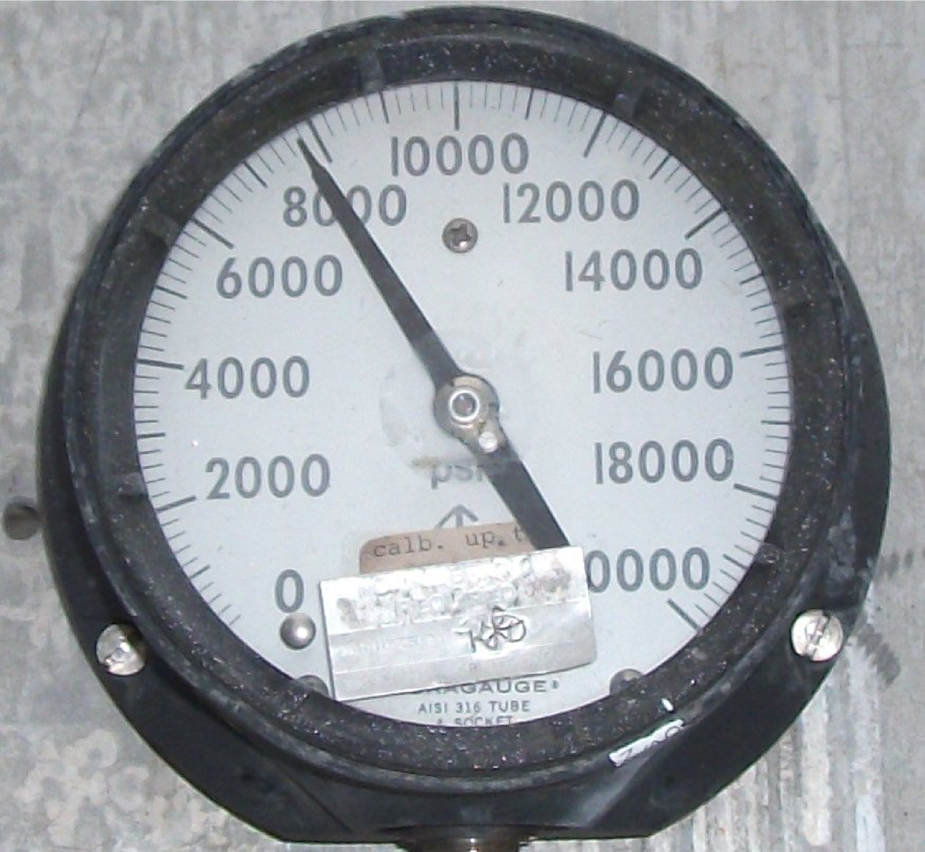How low and how high could this pressure actually be, given the stated accuracy of this gauge?

Reveal answer
• #### Question 3

Interpret the pressure measurement displayed by this gauge mechanism, assuming a gauge accuracy of $\pm$ 0.5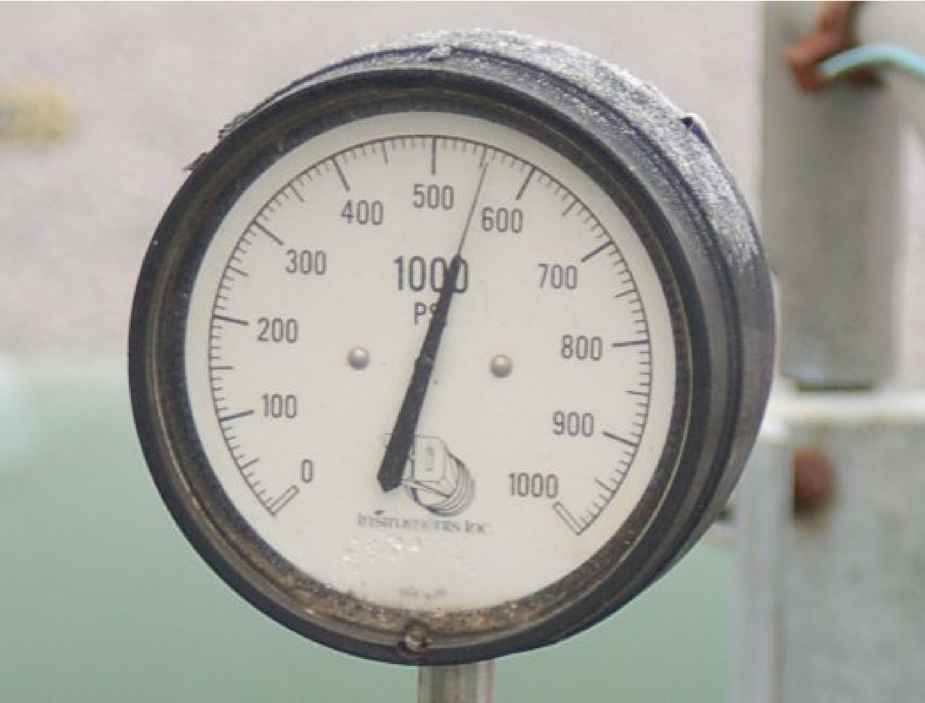How low and how high could this pressure actually be, given the stated accuracy of this gauge?

Reveal answer
• #### Question 4

Interpret the pressure measurement displayed by this gauge mechanism, assuming a gauge accuracy of $\pm$ 2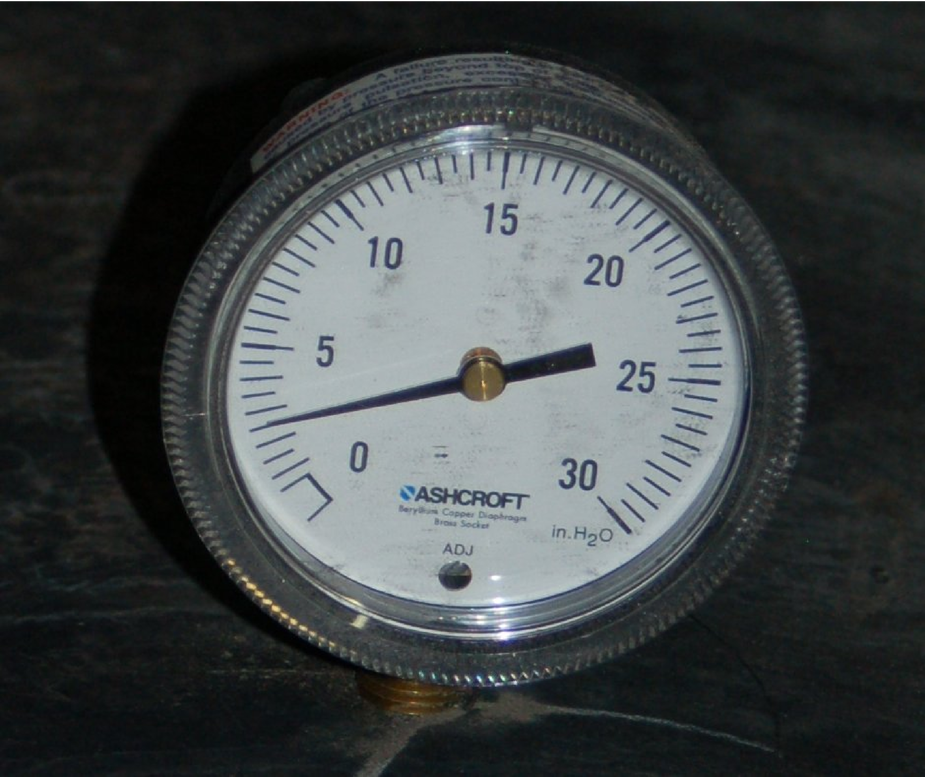How low and how high could this pressure actually be, given the stated accuracy of this gauge?

Reveal answer
• #### Question 5

Interpret the pressure measurement displayed by this gauge mechanism, assuming a gauge accuracy of $\pm$ 2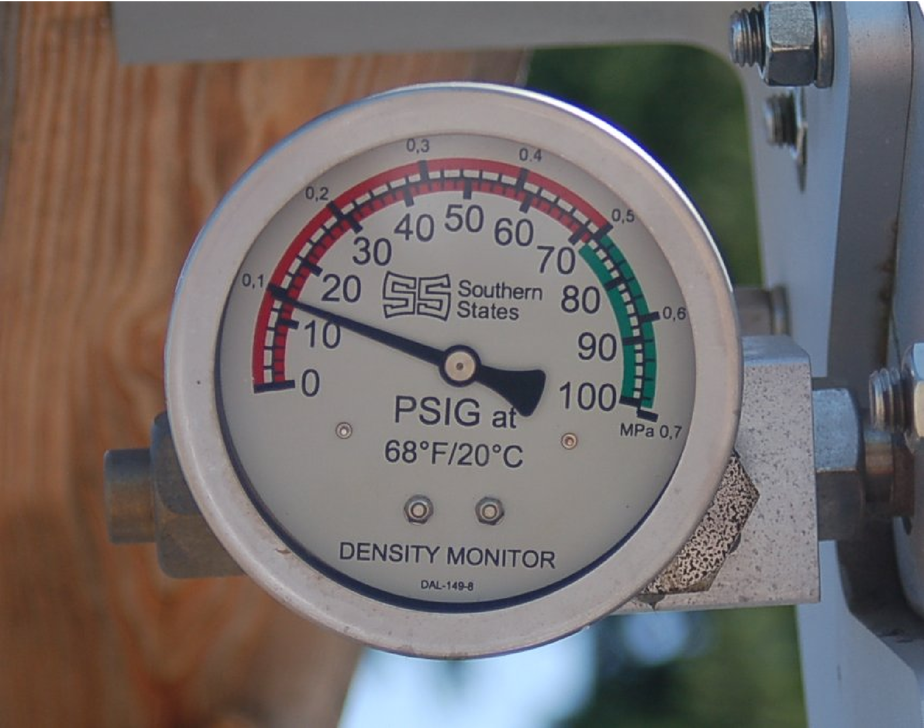How low and how high could this pressure actually be, given the stated accuracy of this gauge?

Reveal answer
• #### Question 6

Interpret the pressure measurement displayed by this U-tube manometer, recalling that the pressure will be equal to the {\it difference} in heights between the two liquid columns: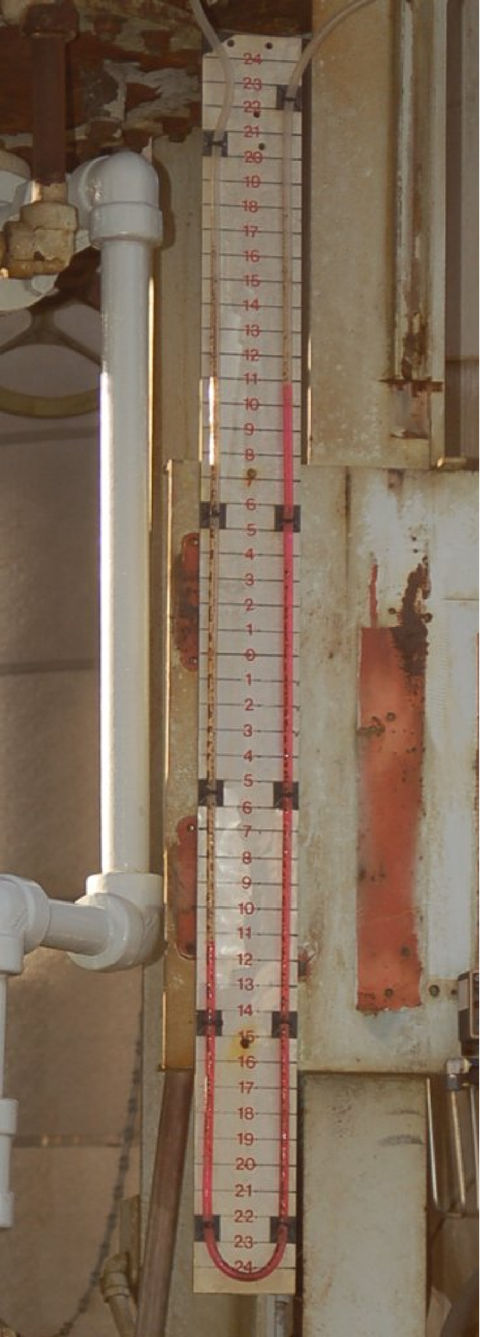Reveal answer
• #### Question 7

Interpret the temperature measurement displayed by this gauge mechanism, and also identify the meaning of the {\it other} pointer: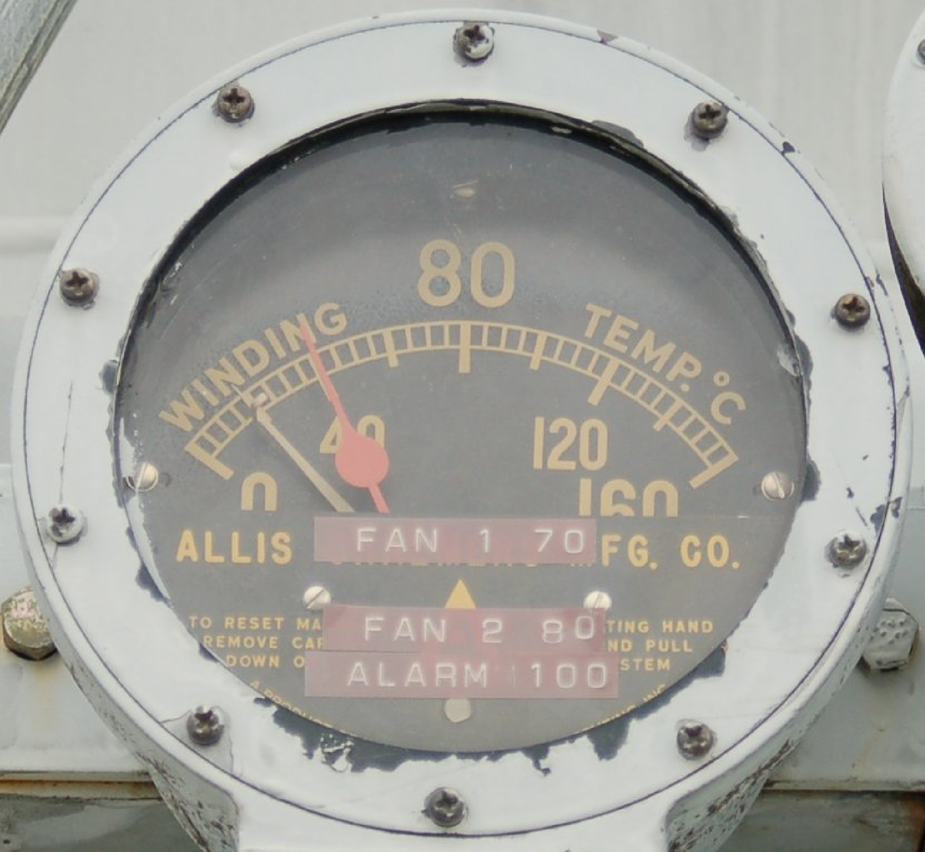Reveal answer
• #### Question 8

Interpret the measurement displayed by this pneumatic controller: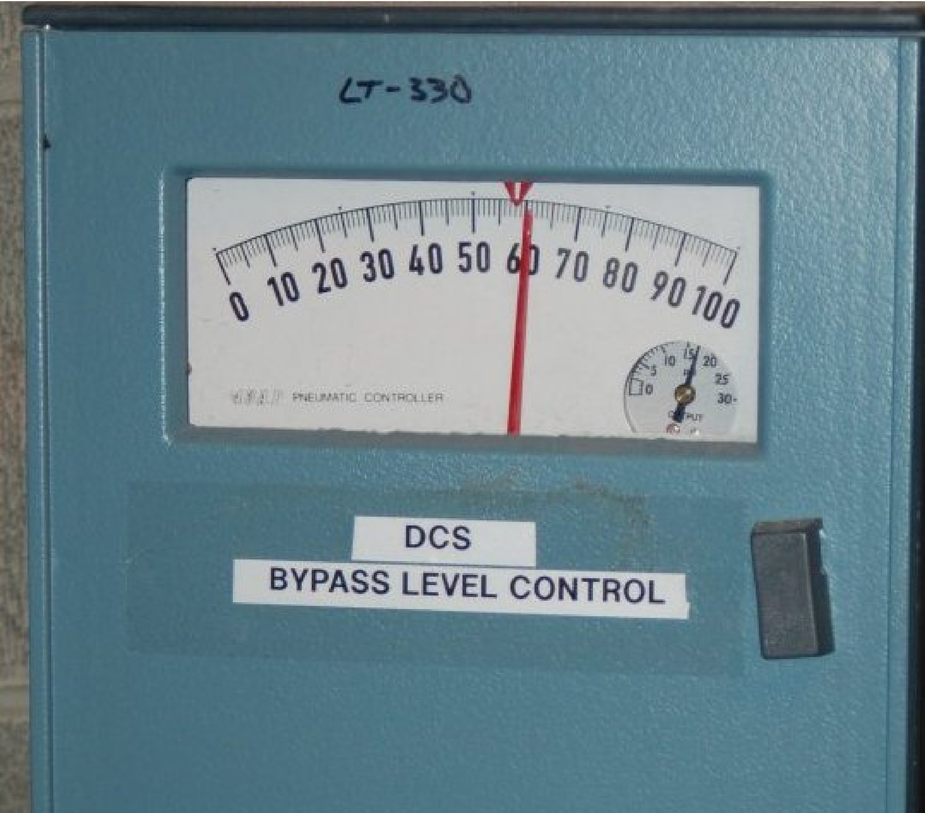Reveal answer
• #### Question 9

Interpret the air flow measurement displayed by this rotameter: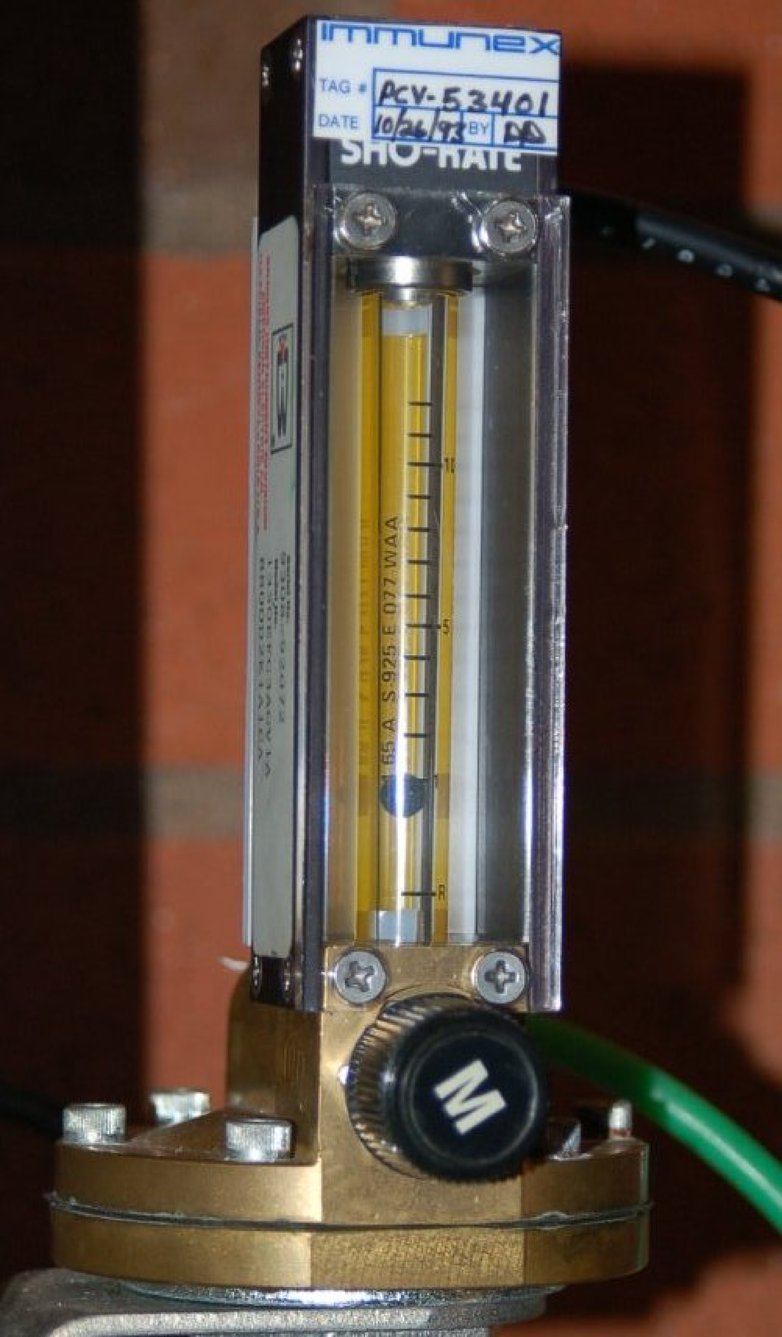Reveal answer
• #### Question 10

Machinists use a tool called {\it calipers} to measure short distances such as the outside diameter of a shaft. One particular type of caliper has a special scale that slides along with the movable jaw called a {\it vernier} scale. The spacing between divisions on the vernier scale is slightly less than the spacing between divisions on the main scale, which means only one division on the vernier scale will ever line up with a division on the main scale at any given time:

In order to obtain a coarse measurement of the caliper’s jow position, read the main scale using the 0’’ mark on the vernier scale as a pointer: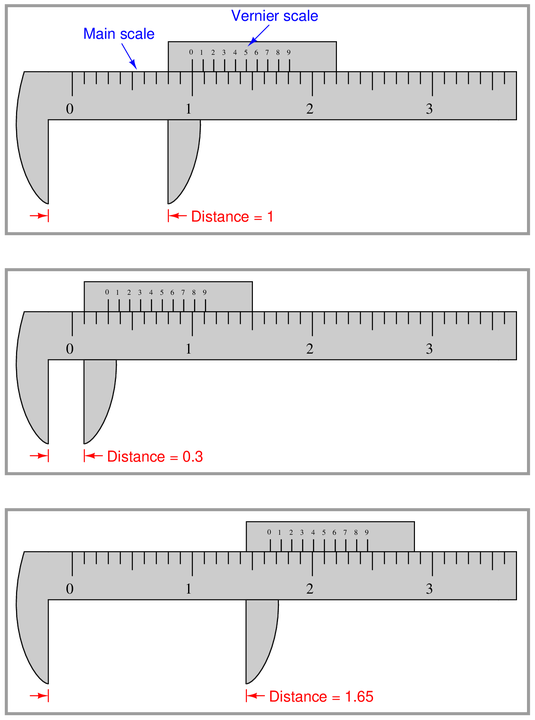Now, use the vernier scale to read the distance between the caliper’s jaws, when the 0’’ division on the vernier scale does {\it not} line up perfectly with one of the divisions on the main scale:Reveal answer
• #### Question 11

Interpret the distance between the jaws of this {\it vernier} caliper: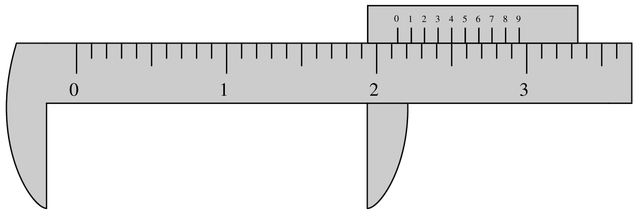Reveal answer
• #### Question 12

Some precision manometers are equipped with a {\it vernier} scale in order to make very precise measurements of liquid column height.

Interpret the reading shown on this mercury manometer. Note: the hairline’’ on the moving vernier scale is adjusted to the meniscus height by a screw prior to taking the reading: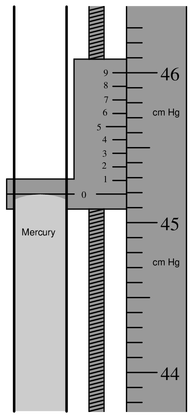Reveal answer

### Related Content

• Share

Published under the terms and conditions of the Creative Commons Attribution License

1 Comment